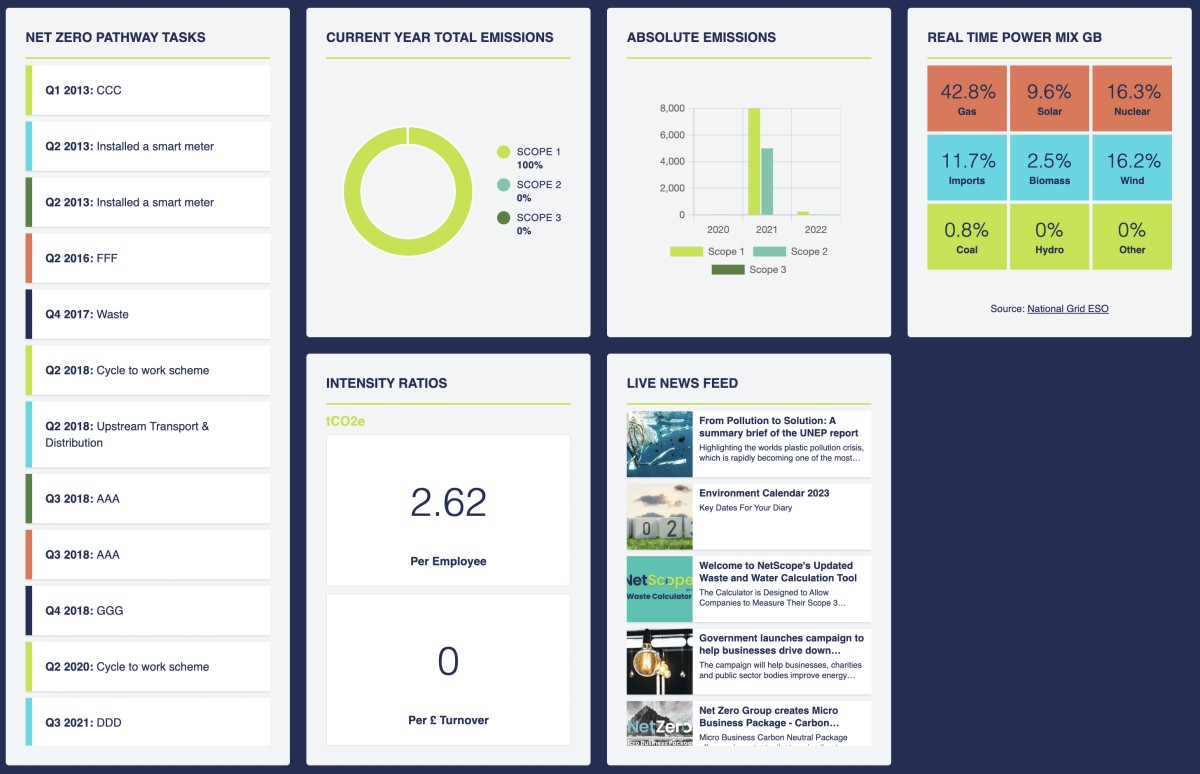26 Jun 2023

# NZG Upgrade NetScope Calculations based on BEIS 2023 Conversion Factor Update

Net Zero Group have updated NetScope to include the BEIS 2023 conversion factors, which were published on 7th June 2023. NetScope users will now be able to seamlessly calculate time-bounded accurate emissions based on the date of input for backdated years. In this process, NZG also updated the NetScope software to include the automated smart selection of conversion factors for footprints backdated to 2019. This new selection process is explained here:

### Standard Scenario

• For calculations covering reporting periods (dated inputs) following 07/06/23, the new 2023 BEIS conversion factors will be selected for all applicable calculations. This is because the BEIS Conversion Factor Update for 2023 has been time-stamped in the platform as 7th June 2023.

• For calculations covering reporting periods (dated inputs) preceding 07/06/23 but after 22/06/22 (the release date of the BEIS 2022 conversion factors), the BEIS 2022 conversion factors will be selected for all applicable calculations.

• Similarly, for all reporting periods backdating to 2019, the conversion factors used in the calculation will be selected based on the associated date of data input, separated by conversion factor update dates for each year.

For calculations with associated dates spanning across the conversion factor update, the following occurs:

### Weighted Average

• In the case where half of the period for the input is in 2022 and half is in 2023; E.g. If Conversion Factor update is 7th June, Electricity bill 1st June - 30th June: 7 days using 2022 conversion factors and 23 days using 2023 conversion factors. Calculation: ((total kWh/30) x 7 x 2022 conversion factor) + ((total kWh/30) x 23 x 2023 conversion factor).

• If the NetScope user was to input, for example, an aggregated annual electricity bill in one input covering every month of the calendar year (1st January 2022 to 31st December 2023), the calculation would work by identifying the kWh per day, and separately multiplying by the respective number of days to be calculated using the BEIS 2022 and BEIS 2023 conversion factors, as follows: